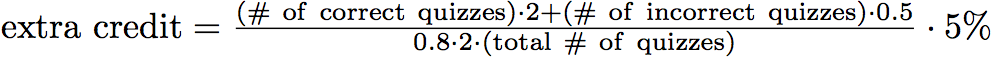## Composition

The final grade in this course will be based on homework, midterm exams, and the final exam. Each of these items will count as follows:
Homework: 30%
Midterm 1: 20%
Midterm 2: 20%
Final Exam: 30%

The final grade in the course will be assigned on a fixed scale as follows:
84% < Grade < 92% --- 3.5
76% < Grade < 84% --- 3.0
68% < Grade < 76% --- 2.5
60% < Grade < 68% --- 2.0
52% < Grade < 60% --- 1.5
44% < Grade < 52% --- 1.0
Completing the in-class clicker questions can earn you up to 5% extra credit. You get 2 points for a correct answer and 0.5 points for an incorrect answer. A total of 80% of the total clicker points you will get the full 5% extra credit. The clicker score is thus calculated according to the following equation:.